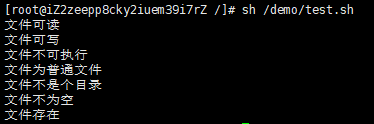# 《前端运维》一、Linux基础–05Shell运算符

今天我们来学习下Shell运算符，Shell跟其他的编程语言一样，也支持多种运算符，包括：

• 算数运算符
• 关系运算符
• 布尔运算符
• 字符串运算符
• 文件测试运算符

#### 一、算数运算符

+ 加法 `expr \$a + \$b` 结果为 30。

* 乘法 `expr \$a \* \$b` 结果为  200。
/ 除法 `expr \$b / \$a` 结果为 2。
% 取余 `expr \$b % \$a` 结果为 0。
= 赋值 a=\$b 将把变量 b 的值赋给 a。
== 相等。用于比较两个数字，相同则返回 true。 [ \$a == \$b ] 返回 false。
!= 不相等。用于比较两个数字，不相同则返回 true。 [ \$a != \$b ] 返回 true。

1. 原生bash是不支持算数运算的，需要通过命令来实现，常用的就是awk和expr，expr更常用于算术运算。
2. 数学运算要用反引号包裹，就像上面例子里的那样。
3. 表达式和运算符之间必须要有空格。
4. 乘号(*)前边必须加反斜杠(\)才能实现乘法运算。

```#!/bin/bash
# 定义变量
a=10
b=20
# 算数运算
val=`expr \$a + \$b`
echo "a + b : \$val"

val=`expr \$a - \$b`
echo "a - b : \$val"

val=`expr \$a \* \$b`
echo "a * b : \$val"

val=`expr \$b / \$a`
echo "b / a : \$val"

val=`expr \$b % \$a`
echo "b % a : \$val"

if [ \$a == \$b ]
then
echo "a 等于 b"
fi
if [ \$a != \$b ]
then
echo "a 不等于 b"
fi```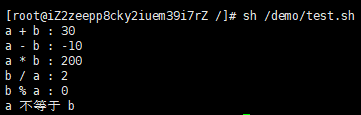哎。。。上面的例子就是抄菜鸟的，一点改动都没有。

#### 二、关系运算符

-eq 检测两个数是否相等，相等返回 true。 [ \$a -eq \$b ] 返回 false。
-ne 检测两个数是否不相等，不相等返回 true。 [ \$a -ne \$b ] 返回 true。
-gt 检测左边的数是否大于右边的，如果是，则返回 true。 [ \$a -gt \$b ] 返回 false。
-lt 检测左边的数是否小于右边的，如果是，则返回 true。 [ \$a -lt \$b ] 返回 true。
-ge 检测左边的数是否大于等于右边的，如果是，则返回 true。 [ \$a -ge \$b ] 返回 false。
-le 检测左边的数是否小于等于右边的，如果是，则返回 true。 [ \$a -le \$b ] 返回 true。

1. 关系运算符只支持数字，不支持字符串，除非字符串也是数字。

```#!/bin/bash
a=10
b=20

if [ \$a -eq \$b ]
then
echo "\$a -eq \$b : a 等于 b"
else
echo "\$a -eq \$b: a 不等于 b"
fi
if [ \$a -ne \$b ]
then
echo "\$a -ne \$b: a 不等于 b"
else
echo "\$a -ne \$b : a 等于 b"
fi
if [ \$a -gt \$b ]
then
echo "\$a -gt \$b: a 大于 b"
else
echo "\$a -gt \$b: a 不大于 b"
fi
if [ \$a -lt \$b ]
then
echo "\$a -lt \$b: a 小于 b"
else
echo "\$a -lt \$b: a 不小于 b"
fi
if [ \$a -ge \$b ]
then
echo "\$a -ge \$b: a 大于或等于 b"
else
echo "\$a -ge \$b: a 小于 b"
fi
if [ \$a -le \$b ]
then
echo "\$a -le \$b: a 小于或等于 b"
else
echo "\$a -le \$b: a 大于 b"
fi```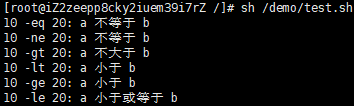#### 三、布尔运算符

! 非运算，表达式为 true 则返回 false，否则返回 true。 [ ! false ] 返回 true。
-o 或运算，有一个表达式为 true 则返回 true。 [ \$a -lt 20 -o \$b -gt 100 ] 返回 true。
-a 与运算，两个表达式都为 true 才返回 true。 [ \$a -lt 20 -a \$b -gt 100 ] 返回 false。

```#!/bin/bash
a=10
b=20

if [ \$a != \$b ]
then
echo "\$a != \$b : a 不等于 b"
else
echo "\$a == \$b: a 等于 b"
fi
if [ \$a -lt 100 -a \$b -gt 15 ]
then
echo "\$a 小于 100 且 \$b 大于 15 : 返回 true"
else
echo "\$a 小于 100 且 \$b 大于 15 : 返回 false"
fi
if [ \$a -lt 100 -o \$b -gt 100 ]
then
echo "\$a 小于 100 或 \$b 大于 100 : 返回 true"
else
echo "\$a 小于 100 或 \$b 大于 100 : 返回 false"
fi
if [ \$a -lt 5 -o \$b -gt 100 ]
then
echo "\$a 小于 5 或 \$b 大于 100 : 返回 true"
else
echo "\$a 小于 5 或 \$b 大于 100 : 返回 false"
fi```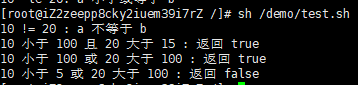#### 四、逻辑运算符

&& 逻辑的 AND [[ \$a -lt 100 && \$b -gt 100 ]] 返回 false
|| 逻辑的 OR [[ \$a -lt 100 || \$b -gt 100 ]] 返回 true

```#!/bin/bash
a=10
b=20

if [[ \$a -lt 100 && \$b -gt 100 ]]
then
echo "返回 true"
else
echo "返回 false"
fi

if [[ \$a -lt 100 || \$b -gt 100 ]]
then
echo "返回 true"
else
echo "返回 false"
fi```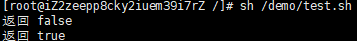#### 五、字符串运算符

= 检测两个字符串是否相等，相等返回 true。 [ \$a = \$b ] 返回 false。
!= 检测两个字符串是否不相等，不相等返回 true。 [ \$a != \$b ] 返回 true。
-z 检测字符串长度是否为0，为0返回 true。 [ -z \$a ] 返回 false。
-n 检测字符串长度是否不为 0，不为 0 返回 true。 [ -n “\$a” ] 返回 true。
\$ 检测字符串是否为空，不为空返回 true。 [ \$a ] 返回 true。

```#!/bin/bash
a="abc"
b="efg"

if [ \$a = \$b ]
then
echo "\$a = \$b : a 等于 b"
else
echo "\$a = \$b: a 不等于 b"
fi
if [ \$a != \$b ]
then
echo "\$a != \$b : a 不等于 b"
else
echo "\$a != \$b: a 等于 b"
fi
if [ -z \$a ]
then
echo "-z \$a : 字符串长度为 0"
else
echo "-z \$a : 字符串长度不为 0"
fi
if [ -n "\$a" ]
then
echo "-n \$a : 字符串长度不为 0"
else
echo "-n \$a : 字符串长度为 0"
fi
if [ \$a ]
then
echo "\$a : 字符串不为空"
else
echo "\$a : 字符串为空"
fi```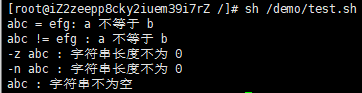#### 六、文件测试运算符

-b file 检测文件是否是块设备文件，如果是，则返回 true。 [ -b \$file ] 返回 false。
-c file 检测文件是否是字符设备文件，如果是，则返回 true。 [ -c \$file ] 返回 false。
-d file 检测文件是否是目录，如果是，则返回 true。 [ -d \$file ] 返回 false。
-f file 检测文件是否是普通文件（既不是目录，也不是设备文件），如果是，则返回 true。 [ -f \$file ] 返回 true。
-g file 检测文件是否设置了 SGID 位，如果是，则返回 true。 [ -g \$file ] 返回 false。
-k file 检测文件是否设置了粘着位(Sticky Bit)，如果是，则返回 true。 [ -k \$file ] 返回 false。
-p file 检测文件是否是有名管道，如果是，则返回 true。 [ -p \$file ] 返回 false。
-u file 检测文件是否设置了 SUID 位，如果是，则返回 true。 [ -u \$file ] 返回 false。
-r file 检测文件是否可读，如果是，则返回 true。 [ -r \$file ] 返回 true。
-w file 检测文件是否可写，如果是，则返回 true。 [ -w \$file ] 返回 true。
-x file 检测文件是否可执行，如果是，则返回 true。 [ -x \$file ] 返回 true。
-s file 检测文件是否为空（文件大小是否大于0），不为空返回 true。 [ -s \$file ] 返回 true。
-e file 检测文件（包括目录）是否存在，如果是，则返回 true。 [ -e \$file ] 返回 true。

```#!/bin/bash
file="/demo/test.sh"
if [ -r \$file ]
then
echo "文件可读"
else
echo "文件不可读"
fi
if [ -w \$file ]
then
echo "文件可写"
else
echo "文件不可写"
fi
if [ -x \$file ]
then
echo "文件可执行"
else
echo "文件不可执行"
fi
if [ -f \$file ]
then
echo "文件为普通文件"
else
echo "文件为特殊文件"
fi
if [ -d \$file ]
then
echo "文件是个目录"
else
echo "文件不是个目录"
fi
if [ -s \$file ]
then
echo "文件不为空"
else
echo "文件为空"
fi
if [ -e \$file ]
then
echo "文件存在"
else
echo "文件不存在"
fi```